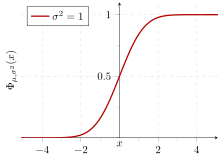## How to Calculate and Solve for Standard Deviation | ProbabilityThe image above represents standard deviation.

To compute for standard deviation, three essential parameters are needed and these parameters are Number of possible outcomes in any single trial (n), Probability of a success in any single trial (p) and Probability of a failure in any single trial (q).

The formula for calculating standard deviation:

σ = √(npq)

Where;

σ = Standard deviation
n = Number of Possible Outcomes in Any Single Trial
p = Probability of a Success in Any Single Trial
q = Probability of a Failure in Any Single Trial

Let’s solve an example;
Find the standard deviation when the number of possible outcomes in any single trial is 14, the probability of a success in any single trial is 0 and the probability of a failure in any single trial is 1.

This implies that;

n = Number of Possible Outcomes in Any Single Trial = 14
p = Probability of a Success in Any Single Trial = 0
q = Probability of a Failure in Any Single Trial = 1

σ = √(npq)
σ = √((14)(0)(1))
σ = √(0)
σ = 0

Therefore, the standard deviation is 0.

Calculating for Number of Possible Outcomes in Any Single Trial when the Standard Deviation, the Probability of a Success in Any Single Trial and the Probability of a Failure in Any Single Trial is Given.

n = σ2 / pq

Where;

n = Number of Possible Outcomes in Any Single Trial
σ = Standard deviation
p = Probability of a Success in Any Single Trial
q = Probability of a Failure in Any Single Trial

Let’s solve an example;
Given that standard deviation is 5, the probability of a success in any single trial is 1 and the probability of a failure in any single trial is 1. Find the number of possible outcomes in any single trial?

This implies that;

σ = Standard deviation = 5
p = Probability of a Success in Any Single Trial = 1
q = Probability of a Failure in Any Single Trial = 1

n = σ2 / pq
n = 52 / 1 x 1
n = 25 / 1
n = 25

Therefore, the number of possible outcomes in any single trial is 25.